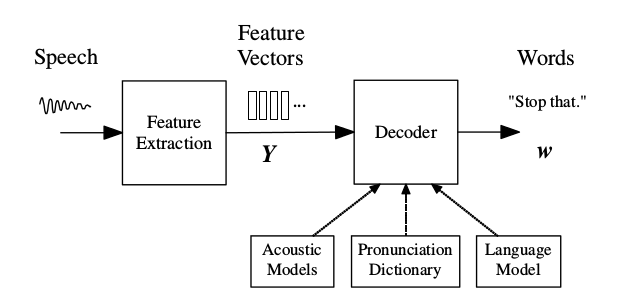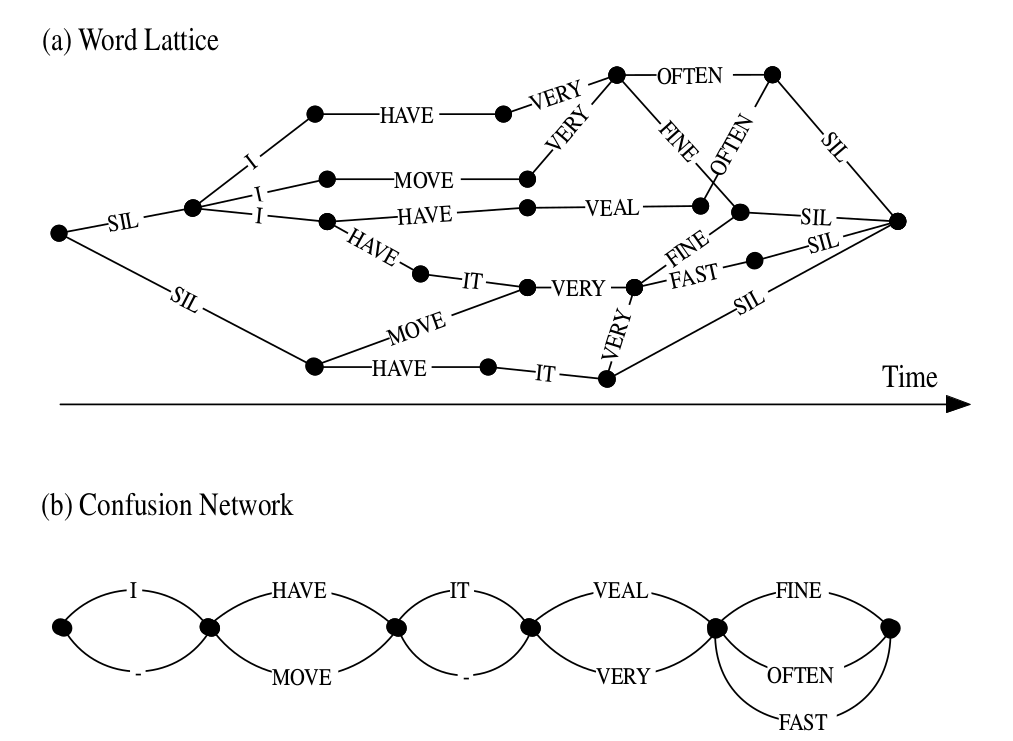# 基于HMM的语音识别(三)

Posted by lili on

## 语音识别各模型的层次结构

$\begin{split} W* & =\underset{W}{argmax}P(W|X) \\ & = \underset{W}{argmax} \frac{P(X|W)P(W)}{P(X)} \\ & = \underset{W}{argmax} P(X|W)P(W) \end{split}$

## 连续语音识别

### 简介

$P(M_i|O) \propto P(O|M_i)P(M_i), \; i=0,1,..,9$

### 音子模型

AQUIRE  AE K W AY R
ABOUTS  AH B AW T S


### 上下文相关的(Context Dependency/CD)音子模型

sil d+oh d-oh+n oh-n+t n-t ah+s ah-s+k s-k sil


sil表示silence，我们可以理解为一个特殊的词，它不考虑上下文。n-t表示当前音子是t而左边是n，ah+s表示当前音子是ah而右边是s。

sil sil-d+oh d-oh+n oh-n+t n-t+ah t-ah+s ah-s+k s-k+sil sil


• 左边是不是一个鼻音？
• 右边是不是摩擦音？

## HMM模型在语音识别系统中的应用

$\hat{w}=\underset{w}{\operatorname{argmax}}{P(w|Y)}$

$\hat{w}=\underset{w}{\operatorname{argmax}}{P(Y|w)P(w)}$图：基于HMM的语音识别系统架构

$P(Y|w)$通过声学模型(Acoustic Model)来确定，而$P(w)$由语言模型来确定。声学模型的基本单元是音子(phone)，比如单词”bat”的音子是/b/ae/t/。

## HMM声学(Acoustic)模型

$P(Y|w)=\sum_Q P(Y|Q) P(Q|w)$

$P(Q|w)=\prod_{l=1}^{L} P(q^{(w_l)}|w_l)$

• 给定前一个时刻的状态，当前状态不依赖更早之前的状态(一阶马尔科夫)
• 给定当前时刻的状态，当前时刻的输出跟其它时刻的无关

$b_j(y)=\mathcal{N}(y;\mu^j,\Sigma^j)=\frac{1}{\sqrt{(2\pi)^n|\Sigma_j|}} e^{-\frac{1}{2} (y_t-\mu_j)^T \Sigma_j^{-1}(y_t-\mu_j)}$

$P(Y|Q)=\sum_{\theta}p(\theta, Y|Q)$

$\begin{split} \theta_1 & =a_0 a_1 a_2 a_2 a_3 a_4/k_0 k_1 k_2 k_3 k_4/ae_0 ae_1 ae_2 ae_3 ae_4/t_0 t_1 t_2 t_3 t_4 \\ \theta_2 & =a_0 a_1 a_2 a_3 a_4/k_0 k_1 k_2 k_3 k_4/ae_0 ae_1 ae_2 ae_2 ae_3 ae_4/t_0 t_1 t_2 t_3 t_4 \end{split}$

$\theta_1$中音素a的中间状态($a_2$)持续了两个时刻，而ae的中间状态$ae_2$只持续了一个时刻；而$\theta_2$正好相反。当然上面我们只列举了两条路径，实际的计算过程中，我们需要变量所有可能的$\theta$，而任意一个状态序列(路径)$\theta_0,\theta_1,…,\theta_{T+1}$(0时刻和T+1时刻是我们增加的进入和退出状态，$\theta_0$跳到$\theta_1$是没有输出的)的概率计算如下：

$p(\theta, Y|Q)=a_{\theta_0 \theta_1}\prod_{t=1}^{T}b_{\theta_t}(y_t)a_{\theta_t \theta_{t+1}}$

$\begin{split} & \hat{\mu}^{(j)}=\frac{\sum_{r=1}^{R} \sum_{t=1}^{T^r} \gamma_t^{(rj)} y_t^{(r)}}{\sum_{r=1}^{R} \sum_{t=1}^{T^r} \gamma_t^{(rj)}} \\ & \hat{\Sigma}^{(j)} = \frac{\sum_{r=1}^{R} \sum_{t=1}^{T^r} \gamma_t^{(rj)} (y_t^{(r)}-\hat{\mu}^{(j)}) (y_t^{(r)}-\hat{\mu}^{(j)})^T}{\sum_{r=1}^{R} \sum_{t=1}^{T^r} \gamma_t^{(rj)}} \end{split}$

$\label{eq:forward-backward2} \hat{a}_{ij} = \frac{\sum_{r=1}^{R} \frac{1}{P^{(r)}} \sum_{t=1}^{T^r} \alpha_t^{(rj)} a_{ij} b_j(y_{t+1}^r) \beta_{t+1}^{(rj)} b_i(y_t^r) }{\sum_{r=1}^{R} \sum_{t=1}^{T^r} \gamma_t^{(rj)}}$

$\begin{split} & \alpha_t^{(rj)} = [\sum_i \alpha_{t-1}^{(ri)} a_{ij}b_j(y_t^{(r)})] \\ & \beta_t^{(ri)} = [\sum_j a_{ij} b_j(y_{t+1}^{(r)}) \beta_{t+1}^{(rj)} ] \end{split}$

$\alpha_1^{(rj)}=a_{0j}b_j(y_1^{(r)})$

$\beta_T^{(ri)}=a_{i,N+1}$

$\gamma_t^{(rj)} = P(\theta_t=s_j|Y^{(r)}; \lambda) = \frac{1}{P^{(r)}} \alpha_t^{(rj)} \beta_t^{(rj)}$

$P^{(r)}=\sum_j \gamma_t^{(rj)}=\sum_j \alpha_t^{(rj)} \beta_t^{(rj)}$图：状态聚类(tied-state)的因子建模

• 首先初始化一个flat-start的monophone的单高斯模型
• 用EM(前向后向)算法估计参数
• 把训练数据的monophone扩展到triphone，把单高斯的monophone模型的参数作为triphone参数的初始值
• 用EM算法重新估计triphone模型的参数
• 使用决策树对triphone的状态进行聚类，然后再用EM算法对聚类后的状态再进行参数重估计

## 语言模型

$P(w)=\prod_{k=1}^{K}P(w_k|w_{k-1}, ..., w_1)$

$P(w)=\prod_{k=1}^{K}P(w_k|w_{k-1},...,w_{k-N+1})$

$\begin{split} H & = - \underset{lim}{K \to \infty} \frac{1}{K} log_2 P(w_1,...,w_K) \\ & \approx \frac{1}{K} \sum_{k=1}^{K} log_2 (P(w_k|w_{k-1},...,w_{k-N+1})) \end{split}$

N-Gram语言模型可以通过最大似然方法来估计参数，假设$C(w_{k−2} w_{k−1} w_k)$表示3个词$(w_{k−2} w_{k−1} w_k$连续出现在一起的次数，类似的$C(w_{k−2} w_{k−1}$表示两个词$w_{k−2} w_{k−1}$连续出现在一起的次数。那么：

$P(w_k|w_{k-1}w_{k-2})=\frac{C(w_{k−2} w_{k−1} w_k)}{C(w_{k−2} w_{k−1})}$

$P(w_k|w_{k-1}, w_{k-2}) = \begin{cases} d \frac{C(w_{k−2} w_{k−1} w_k)}{C(w_{k−2} w_{k−1})} & if 0 < C(w_{k−2} w_{k−1} w_k) \le C' \\ \frac{C(w_{k−2} w_{k−1} w_k)}{C(w_{k−2} w_{k−1})} & if C(w_{k−2} w_{k−1} w_k) > C' \\ \alpha(w_{k-2}, w_{k-1})P(w_k|w_{k-1}) & \text{其它情况} \end{cases}$

## 解码器(Decoder)图：word lattice和confusion network